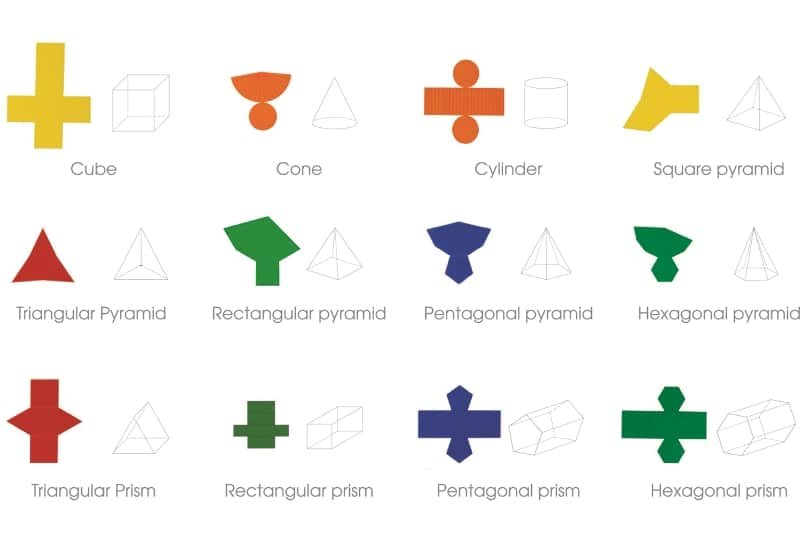# Geometric Models / Solids

#### • Measurement: Surface / Area, Volume / Capacity

Students need to handle 3D objects, turn them, spin them, fill them and cover them in order to fully understand the features of them. This is impossible to emulate on a screen.

Sets of Geometric models vary, but most should include prisms and the associated pyramid. For example, a triangular prism should be linked with a triangular pyramid, a cube with a square based prism, a cylinder with a cone and so on.

### Mathematical Language

Students will need to be taught the names of various 3D objects. The general name given to 3D objects is ‘polyhedra’, which is made up of the words poly (many) and hedra (base). A single 3D object is a polyhedron.

Other language: Apex, base, cone, cube, cylinder, edges, faces, hemisphere, rectangular, hexagonal, pentagonal prisms and pyramids, sphere, square, vertex (singular) and vertices (plural).

#### Others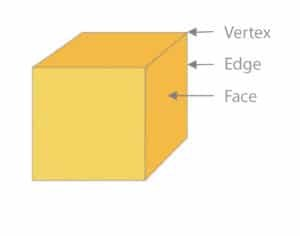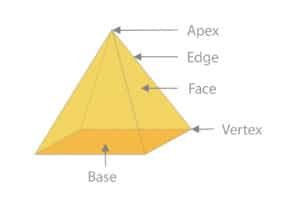### Using Geometric Models / Solids

Geometric models may be used to learn about Eulers’ Law

Vertices + Faces = Edges + 2, V + F = E + 2

Some kits come with folding nets so students may learn about 2D nets and the related 3D solid. The 2D Net will also support the development of surface area understanding.  Some sets are hollow and can be filled to show relationships in terms of the volume and capacity of objects.

#### Surface Area

The surface area of a pyramid involves calculating the area of the base and then the area of the triangular faces.

Some students have trouble visualising all of the faces of 3D solids and find it easier to calculate the surface area using a net.

#### Nets

• A net is the flat 2D pattern that may be folded to make a 3D object. One net for a cube is: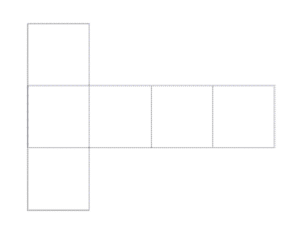• A net for a square based pyramid is
shown here: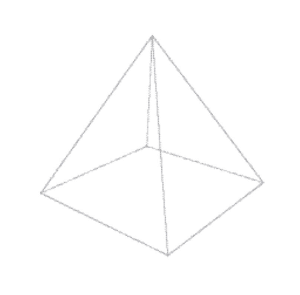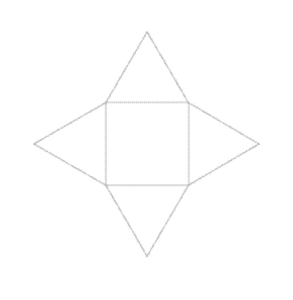### Typical Classroom Requirements

#### A class set:

One set of Geometric Solids can be used for classroom demonstrations. If working in small groups, with students rotating through various task centres then one set would be sufficient. If working as a whole class then one set per four students would be ideal.

### Support and Complementary Materials